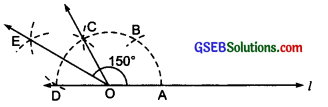# GSEB Solutions Class 6 Maths Chapter 14 Practical Geometry InText Questions

Gujarat Board GSEB Textbook Solutions Class 6 Maths Chapter 14 Practical Geometry InText Questions and Answers.

## Gujarat Board Textbook Solutions Class 6 Maths Chapter 14 Practical Geometry InText Questions

Try These (Page 286)

Question 1.
In Step II of the construction using ruler and compasses, (NCERT Textbook page 285) what would happen if we take the length of radius to be smaller than half the length of $$\overline{\mathrm{AB}}$$?
Solution:
In case we take the radius smaller than half of the length of $$\overline{\mathrm{AB}}$$, the arcs will not intersect each other at two points P and Q.

Try These (Page 289)

Question 1.
In Step II (See NCERT Page 289) above, what would happen if we take radius to be smaller than half the length BC?
Solution:
If we take the radius to be smaller than half of BC, the arcs drawn with centres B and C will not intersect each other.Try These (Page 290)

Question 1.
How will you construct a 15° angle?
Solution:
Steps of construction:
Step I: Construct an angle ABC of 60°.
Step II: Bisect ∠ABC to get an angle of 30°, i.e. ∠ABD = 30°.Step III: Bisect ∠ABD, such that $$\overrightarrow{\mathrm{BE}}$$ is bisector of ∠ABD.
Thus, ∠ABE = $$\frac { 1 }{ 2 }$$(30°) = 15°.

Try These (Page 291)

Question 1.
How will you construct a 150° angle?
Solution:
Steps of construction:
Step I: Draw a line l and mark a point O on it.
Step II: With centre O and a convenient radius, draw an arc intersecting l at A.
Step III: With the same radius and centre at A, draw an arc to cut the first arc at B.
Step IV: Again with the same radius and centre at B, draw another arc to intersect the first arc at C.Step V: Once again with the same radius and centre at C, draw an arc to cut the first arc at D.
Step VI: Now, bisect ∠COD, such that ∠COE = ∠EOD = 30°
Step VII: Since, 150° = 120° + 30°, therefore ∠AOC + ∠COE = ∠AOE. Thus, ∠AOE is the required angle Whose measure is 150°Try These (Page 291)

Question 1.
How will you construct a 45° angle?
Solution:
Steps of construction:
Step I: Construct an angle of 90° as shown in the figure. ∠POQ = 90°.Step II: Draw OR,the angle bisector of ∠POQ such that
$$\frac { 1 }{ 2 }$$[∠POQ] = $$\frac { 1 }{ 2 }$$(90°) = 45°
or ∠POQ = 45°